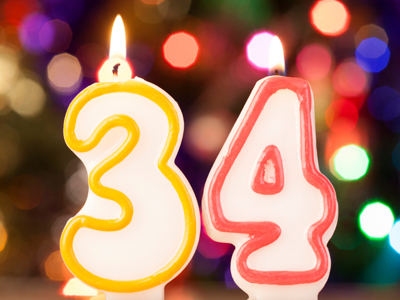The best method of multiplying 34 by 4 is to double and double again.

# Multiplication and Division (Year 4)

In Year Four Maths KS2 students continue to look at multiplication and division. As well as learning their times tables they will also start to multiply and divide larger numbers. Terms such as 'product' and 'remainder' should be familiar to children in Year Four and they should also be able to find simple fractions, such as halves or quarters, of other numbers.

If you want to find the product of two numbers then multiply them together. The numbers that we can divide a number by are it's factors. Products and factors are just two parts of multiplication and division. Multiplication and division are often used by people in their everyday life, especially when going shopping! Three children have broken their toothbrushes so their Mum is buying three new ones. They cost £4 each, so to find the total she has to multiply £4 by 3. If Mum knows the answer then she knows just how much she is spending.

Do you know what the product of two numbers is? Can you multiply and divide? See how much you know about multiplication and division by playing this quiz.

1.
12 x 0 = ?
0
1
6
12
Any number multiplied by 0 will give the answer 0
2.
How do you find half of a number?
Multiply it by 4
Divide it by 2
Multiply it by 2
Divide it by 4
Half means the same as divided by 2
3.
Which is equivalent to multiply by 10 then double?
x 5
x 15
x 20
x 25
Remember that double means the same as x 2, so 10 x 2 = 20
4.
What is the product of 3 and 6?
2
3
9
18
To find the product of two numbers they have to be multiplied together
5.
If we divide 10 by 4 what is the answer?
2
2 remainder 2
It can’t be done
3
2 x 4 = 8 so there are 2 numbers left over
6.
Which times table would you have if you doubled the 4 times table?
The 2 times table
The 3 times table
The times table
The 8 times table
2 x 4 = 8
7.
How do you find quarter of a number?
Multiply it by 4
Divide it by 2
Multiply it by 2
Divide it by 4
Quarter means the same as divided by 4
8.
What is the best method of multiplying 34 by 4?
Double and double again
Multiply by 3 twice
Multiply by 3 then halve
Multiply by 2 then halve
Double twice to multiply any number by 4
9.
Which strategy would be best to multiply 87 by 5?
Multiply by 4 then add another 87
Double then multiply by 3
Multiply by 10 then halve
Multiply by 3 then halve
10 and 2 are easier numbers to deal with than 5 is
10.
Which of these is not equivalent to 64 x 3?
64 + 64 + 64
3 x 64
6 x 64 x 2
64 x 2 + 64
The correct answer is 192 but 6 x 64 x 2 = 768